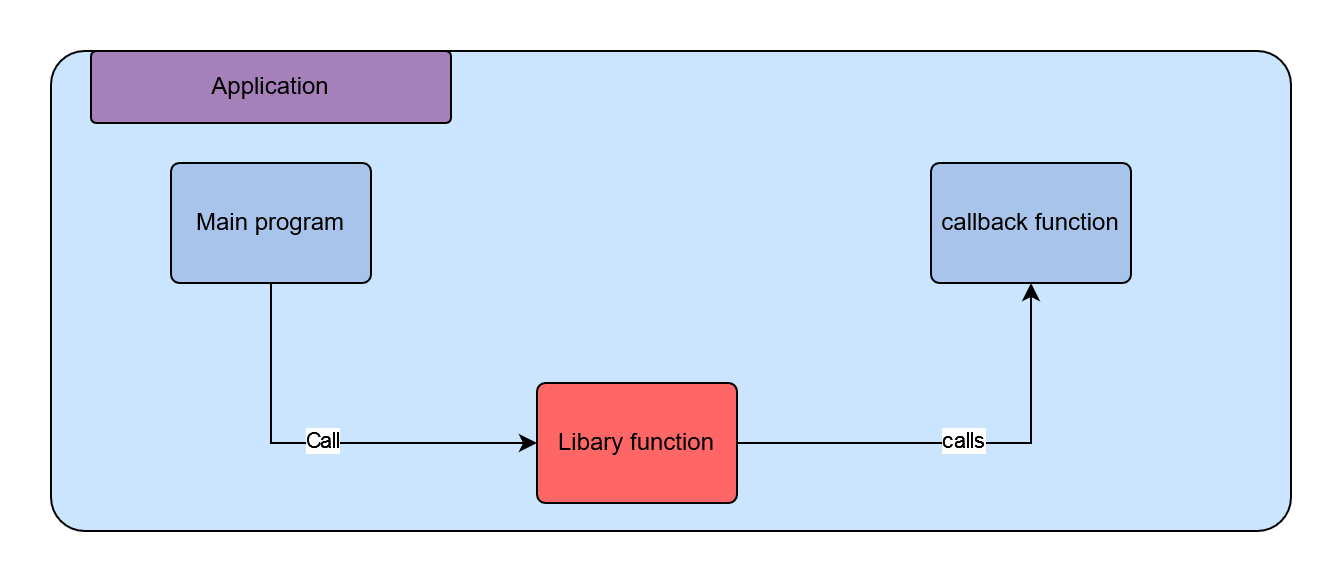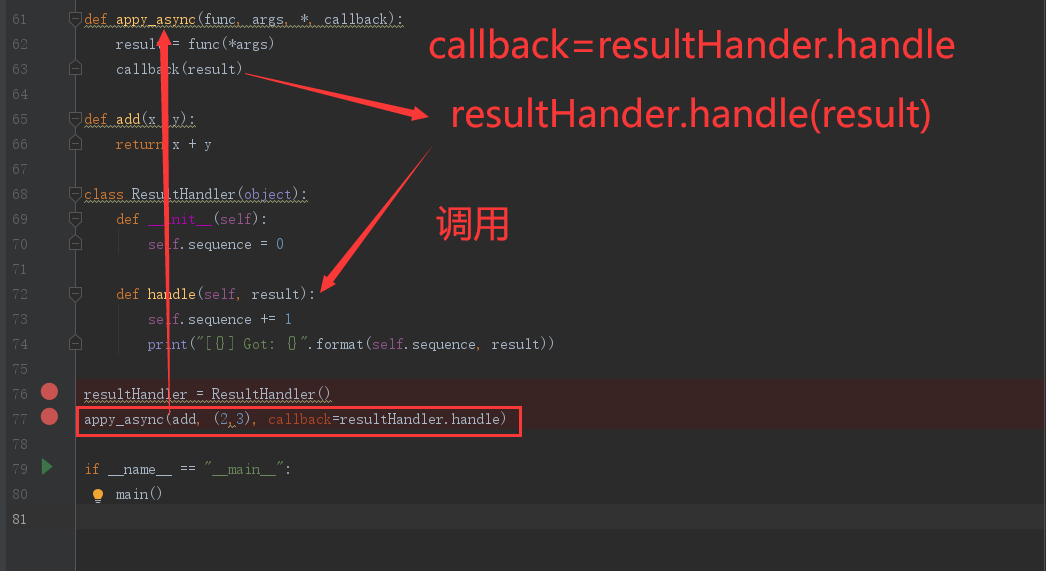# 什么是回调函数

## 例如：# 回调机制的优势

``#回调函数1#生成一个2k形式的偶数def double(x):    return x * 2    #回调函数2#生成一个4k形式的偶数def quadruple(x):    return x * 4 callback_demo.py` from even import * #中间函数#接受一个生成偶数的函数作为参数#返回一个奇数def getOddNumber(k, getEvenNumber):    return 1 + getEvenNumber(k)    #起始函数，这里是程序的主函数def main():        k = 1    #当需要生成一个2k+1形式的奇数时    i = getOddNumber(k, double)    print(i)    #当需要一个4k+1形式的奇数时    i = getOddNumber(k, quadruple)    print(i)    #当需要一个8k+1形式的奇数时    i = getOddNumber(k, lambda x: x * 8)    print(i)    if __name__ == "__main__":    main()``

# 异步处理有关的回调函数

``def apply_ascyn(func, args, callback):    """     func 函数的是处理的函数    args 表示的参数    callback 表示的函数处理完成后的 该执行的动作    """    result = func(*args)    callback(result) def add(x, y):    return x + y def print_result(result):    print(result) apply_ascyn(add, (2, 3), callback=print_result)``

## 使用一个绑定方法来代替这个简单函数。

``def appy_async(func, args, *, callback):    result = func(*args)    #异步执行的函数 同时将执行后返回到这个早从这个函数跳出去    callback(result) def add(x ,y):    return x + y class ResultHandler(object):    def __init__(self):        self.sequence = 0     def handle(self, result):        self.sequence += 1        print("[{}] Got: {}".format(self.sequence, result)) resultHandler = ResultHandler()appy_async(add, (2,3), callback=resultHandler.handle)``## 使用闭包代替上面的类来实现

``def apply_async(func, args, *, callback):    result = func(*args)    callback(result) def add(x ,y):    return x + y def make_handler():    sequence = 0    def handler(result):        nonlocal sequence        sequence += 1        print("[{}] Got:{}".format(sequence, result))    return handler handler = make_handler()apply_async(add, (2,3), callback=handler)``

## 使用协程来完成异步操作

``def apply_async(func, args, *, callback):    result = func(*args)    callback(result) def add(x, y):    return x + y def make_handler():    sequence = 0    while True:        result = yield        sequence += 1        print("[{}] Got:{}".format(sequence, result)) handle = make_handler()next(handle) apply_async(add, (2,3), callback=handle.send)``

python3回调函数(callback) – 知乎